Page 1 of 1

RF Designer - Components (Universal Elements)

Posted: Sat Feb 07, 2015 10:54 pm
Universal Elements

GAIN - Ideal Gain Block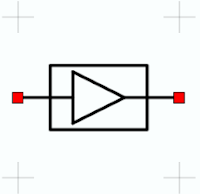Name Description Units Default Value A Gain decibels 0.0 dB SL Positive gain slope dB/octave 6.0 dB/octave SH Negative gain slope dB/octave 6.0 dB/octave FL Lower frequency for SL Frequency 0.0 MHz FH Upper frequency for SH Frequency 0.0 MHz R Reference impedance Resistance 50 ohms

Model for an ideal gain block. Gain increases to the user defined gain (G) at the use defined lower frequency (FL) at a slope determined by the positive gain slope (SL). The gain stays constant until the user defined upper frequency (FH) at which point, gain will decrease at a rate defined by the negative gain slope (SH). The upper or lower gain slopes can be omitted by setting FL/FH or SL/SH to 0.

CIRC - Ideal Circulator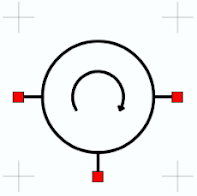Model for an ideal universal 3 port circulator. This model is frequency independent.

ISOL - Ideal Isolator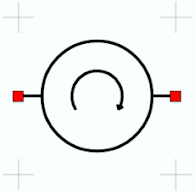Model for an ideal universal 2 port isolator. This model is frequency independent.

CPLR - Ideal Coupler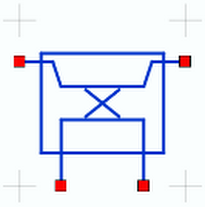Name Description Units Default Value c Coupling coefficient unitless 0.1

Model for an ideal coupler. Coupling is determined by the coupling coefficient (c). Coupling in decibels is calculated as 20*log(c). This model is frequency independent.

GYR - Gyrator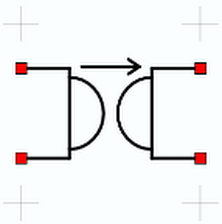Name Description Units Default Value R Gyrational resistance Resistance 50 ohms

Model for an ideal gyrator. This model is frequency independent.